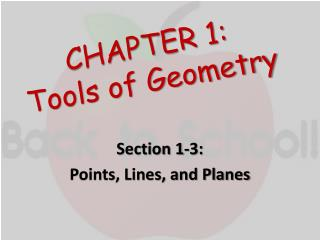DownloadDownload PresentationCHAPTER 1: Tools of Geometry

# CHAPTER 1: Tools of Geometry

Télécharger la présentation## CHAPTER 1: Tools of Geometry

- - - - - - - - - - - - - - - - - - - - - - - - - - - E N D - - - - - - - - - - - - - - - - - - - - - - - - - - -
##### Presentation Transcript

1. CHAPTER 1:Tools of Geometry Section 1-3: Points, Lines, and Planes

2. Objectives • To understand basic terms of geometry. • To understand basic postulates of geometry.

3. Vocabulary • Point • Space • Line • Collinear Points • Plane • Coplanar • Postulate • Axiom

4. Point • A point is a location. • A point has no size. • Represented by a small dot and capital letter. • Geometric figures consist of sets of points.

5. Space • Space is defined as the set of all points.

6. Line • A line is a series of points that extends in two opposite directions without end. • Ways to name a line: • Use any two points on the line. • A single lowercase italics letter.

7. Collinear Points • Points that lie on the same line are collinear points.

8. Identifying Collinear Points • In the diagram, are E, F, and C collinear? • Are E, F, and D collinear?

9. Plane • A plane is a flat surface that has no thickness. • A plane contains many lines and extends without end in the direction of all of its lines. • Naming a plane: • You may use a single capital letter. • You may use three or more of its non-collinear points.

10. Postulate (or Axiom) • A postulate (or axiom) is an accepted statement of fact.

11. Postulate 1-1: • Through any two points, there is exactly one line.

12. Postulate 1-2: • If 2 lines intersect, they intersect in exactly one point.

13. Postulate 1-3: • If two planes intersect, they intersect in exactly one line.

14. Finding the intersection of two planes. • Where do planes ABC and plane DZY intersect? W Z A D X Y B C

15. Postulate 1-4: • Through any three noncollinear points, there is exactly one plane.